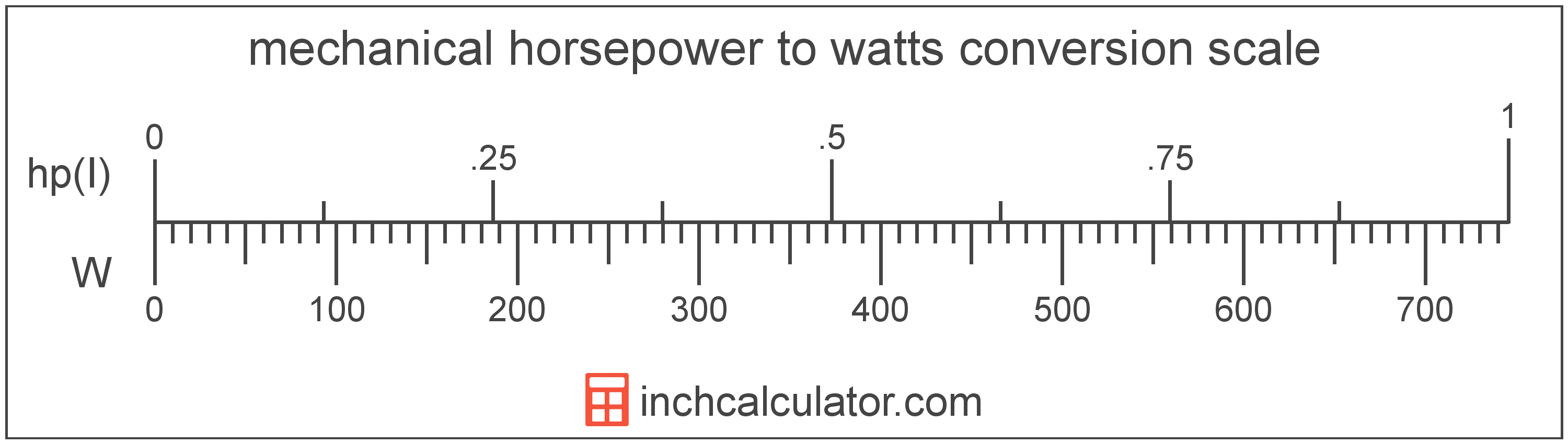# Convert Mechanical Horsepower to Watts

Enter the power in mechanical horsepower below to get the value converted to watts.

Results in Watts:1 hp(I) = 745.699872 W

## How to Convert Mechanical Horsepower to WattsTo convert a mechanical horsepower measurement to a watt measurement, multiply the power by the conversion ratio. One mechanical horsepower is equal to 745.699872 watts, so use this simple formula to convert:

watts = mechanical horsepower × 745.699872

The power in watts is equal to the mechanical horsepower multiplied by 745.699872.

For example, here's how to convert 5 mechanical horsepower to watts using the formula above.
5 hp(I) = (5 × 745.699872) = 3,728.499358 W

Mechanical horsepower and watts are both units used to measure power. Keep reading to learn more about each unit of measure.

## Mechanical Horsepower

Mechanical horsepower is often used to measure the output of engines and motors in North America. One mechanical horsepower is equal to 745.69987158227022 watts.

The mechanical horsepower is a US customary and imperial unit of power. Mechanical horsepower can be abbreviated as hp(I), for example 1 mechanical horsepower can be written as 1 hp(I).

Try our engine horsepower calculator to calculate the power of a motor.

## Watts

One watt is the power equal to the rate of energy of one joule per second in an electrical circuit. One watt is also equal to the power produced in a circuit with a voltage potential of one volt with a current of one amp.

The watt is the SI derived unit for power in the metric system. Watts can be abbreviated as W, for example 1 watt can be written as 1 W.

Watt's Law states the relationship between power, current, and voltage. Using Watt's Law, it's possible to express the power in watts as an expression using current and voltage.

PW = IA × VV

The power in watts is equal to the current in amperes times the potential difference in volts.

Combining this with Ohm's Law, it's also possible to express the power in watts using resistance in addition to volts and amps.

## Mechanical Horsepower Measurements and Equivalent Watt Conversions

Common mechanical horsepower values converted to the equivalent watt value
Mechanical Horsepower Watts
1 hp(I) 745.7 W
2 hp(I) 1,491 W
3 hp(I) 2,237 W
4 hp(I) 2,983 W
5 hp(I) 3,728 W
6 hp(I) 4,474 W
7 hp(I) 5,220 W
8 hp(I) 5,966 W
9 hp(I) 6,711 W
10 hp(I) 7,457 W
11 hp(I) 8,203 W
12 hp(I) 8,948 W
13 hp(I) 9,694 W
14 hp(I) 10,440 W
15 hp(I) 11,185 W
16 hp(I) 11,931 W
17 hp(I) 12,677 W
18 hp(I) 13,423 W
19 hp(I) 14,168 W
20 hp(I) 14,914 W
21 hp(I) 15,660 W
22 hp(I) 16,405 W
23 hp(I) 17,151 W
24 hp(I) 17,897 W
25 hp(I) 18,642 W
26 hp(I) 19,388 W
27 hp(I) 20,134 W
28 hp(I) 20,880 W
29 hp(I) 21,625 W
30 hp(I) 22,371 W
31 hp(I) 23,117 W
32 hp(I) 23,862 W
33 hp(I) 24,608 W
34 hp(I) 25,354 W
35 hp(I) 26,099 W
36 hp(I) 26,845 W
37 hp(I) 27,591 W
38 hp(I) 28,337 W
39 hp(I) 29,082 W
40 hp(I) 29,828 W

## More Mechanical Horsepower Power Conversions

SI Units
Convert to Microwatts
1 hp(I) is equal to 745,699,871.6 microwatts
Convert to Milliwatts
1 hp(I) is equal to 745,699.9 milliwatts
Convert to Kilowatts
1 hp(I) is equal to 0.7457 kilowatts
Convert to Megawatts
1 hp(I) is equal to 0.000746 megawatts
US Customary & Imperial Units
Convert to Electric Horsepower
1 hp(I) is equal to 0.999598 electric horsepower
Convert to Btu Per Hour
1 hp(I) is equal to 2,544.43 btu per hour
Other Units
Convert to Kilocalories Per Hour
1 hp(I) is equal to 641.615691 kilocalories per hour